Visualisation Recipe for 'modelbased' Objects

## Usage

# S3 method for estimate_grouplevel
visualisation_recipe(
x,
hline = NULL,
pointrange = NULL,
facet_wrap = NULL,
labs = NULL,
...
)

# S3 method for estimate_means
visualisation_recipe(
x,
show_data = "jitter",
point = NULL,
jitter = point,
boxplot = NULL,
violin = NULL,
line = NULL,
pointrange = NULL,
labs = NULL,
...
)

# S3 method for estimate_predicted
visualisation_recipe(
x,
show_data = "points",
point = NULL,
density_2d = NULL,
line = NULL,
ribbon = NULL,
labs = NULL,
...
)

# S3 method for estimate_slopes
visualisation_recipe(
x,
hline = NULL,
line = NULL,
pointrange = NULL,
ribbon = NULL,
labs = NULL,
facet_wrap = NULL,
...
)

## Arguments

x

A modelbased object.

...

Other arguments passed to other functions.

show_data

Display the "raw" data as a background to the model-based estimation. Can be set to "none" to remove it. When input is the result of estimate_means, show_data can be "points" (the jittered observation points), "boxplot", "violin" a combination of them (see examples). When input is the result of estimate_response or estimate_relation, show_data can be "points" (the points of the original data corresponding to the x and y axes), "density_2d", "density_2d_filled", "density_2d_polygon" or "density_2d_raster".

point, jitter, boxplot, violin, pointrange, density_2d, line, hline, ribbon, labs, facet_wrap

Additional aesthetics and parameters for the geoms (see customization example).

## Examples

# ==============================================
# estimate_grouplevel
# ==============================================
if (require("see") && require("lme4")) {
data <- lme4::sleepstudy
data <- rbind(data, data)
data$Newfactor <- rep(c("A", "B", "C", "D")) # 1 random intercept model <- lmer(Reaction ~ Days + (1 | Subject), data = data) x <- estimate_grouplevel(model) layers <- visualisation_recipe(x) layers plot(layers) }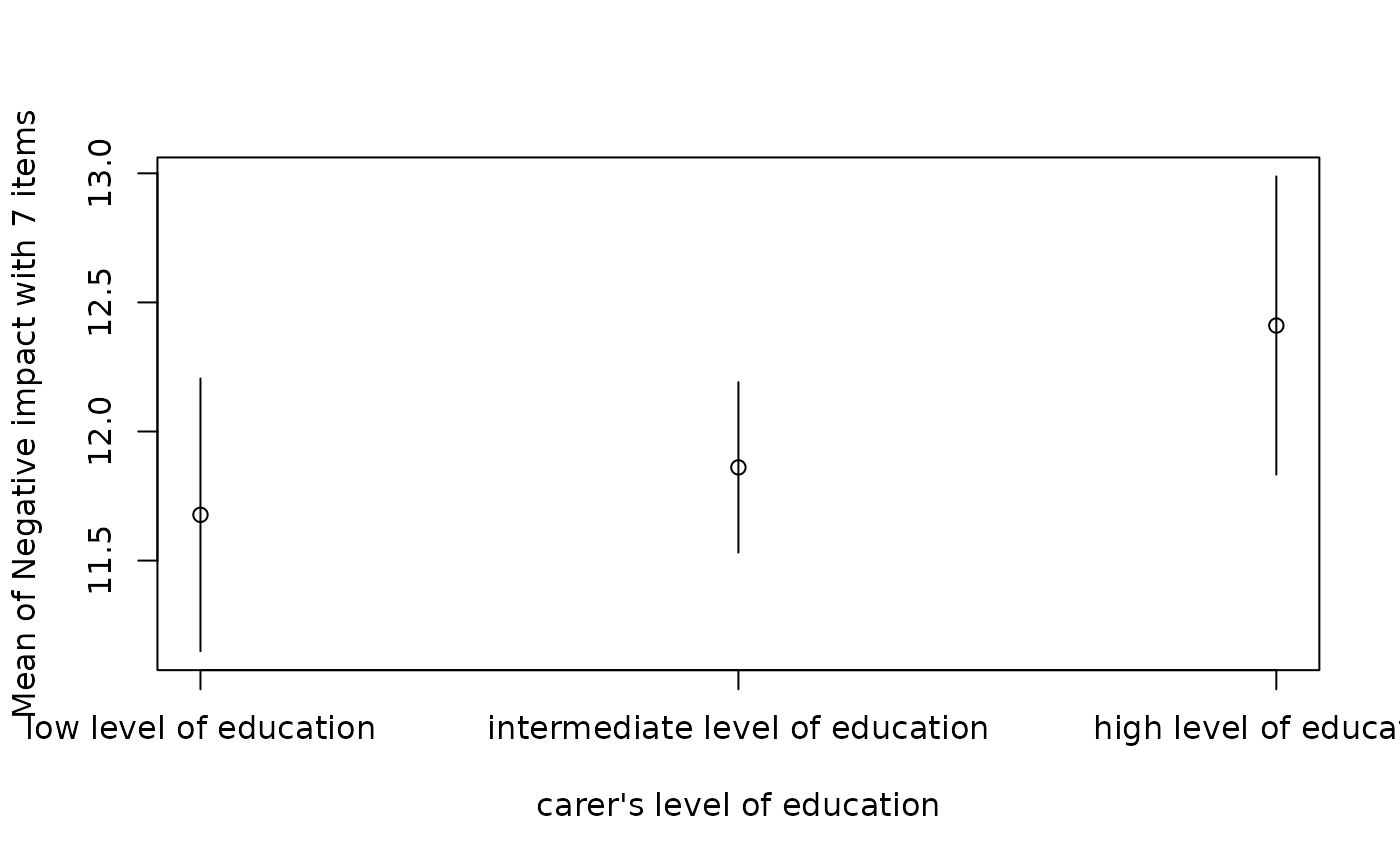# \donttest{ if (require("see") && require("lme4")) { # 2 random intercepts model <- lmer(Reaction ~ Days + (1 | Subject) + (1 | Newfactor), data = data) x <- estimate_grouplevel(model) plot(visualisation_recipe(x)) model <- lmer(Reaction ~ Days + (1 + Days | Subject) + (1 | Newfactor), data = data) x <- estimate_grouplevel(model) plot(visualisation_recipe(x)) }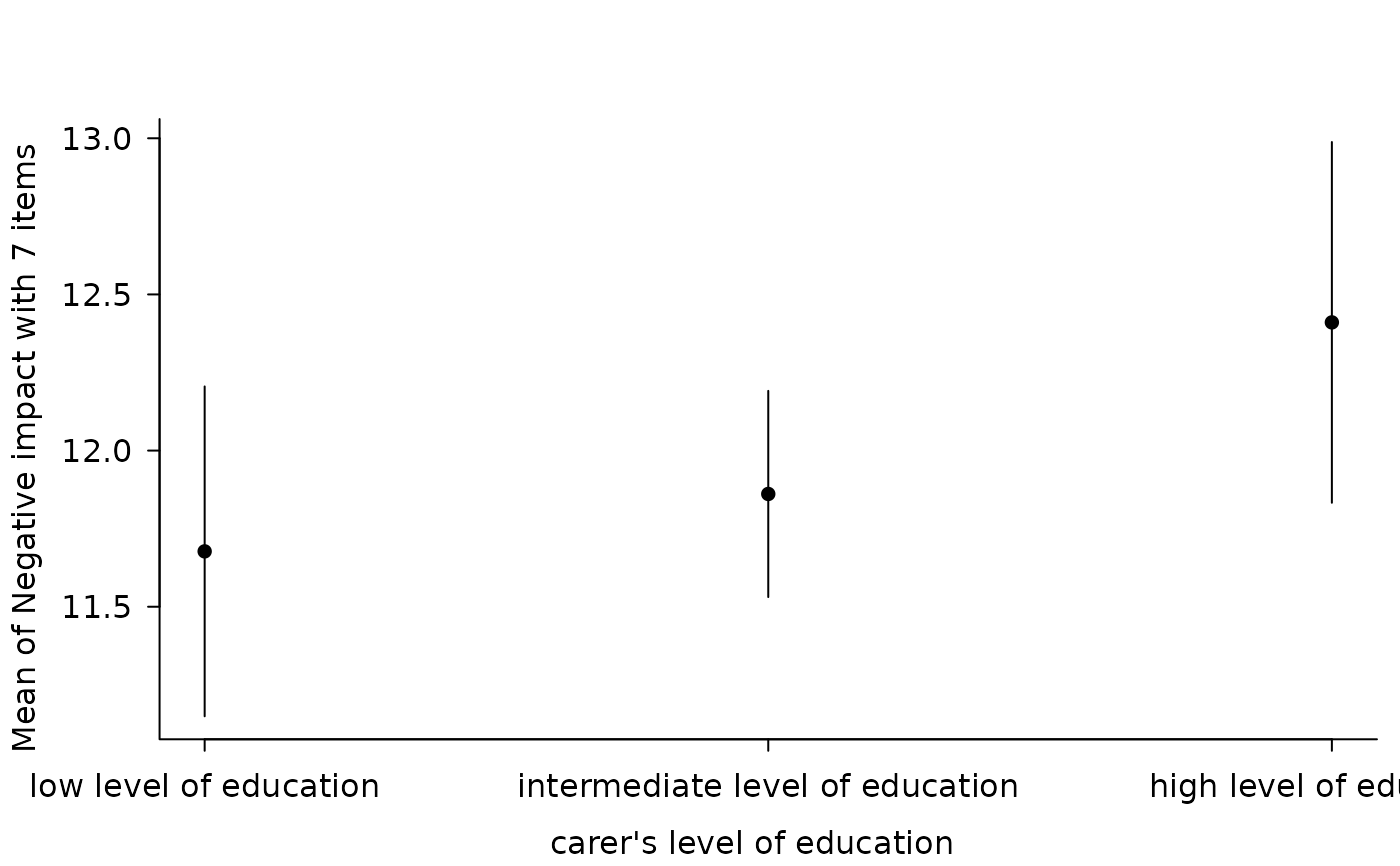# } # Simple Model --------------- x <- estimate_means(lm(Sepal.Width ~ Species, data = iris)) #> We selected at = c("Species"). layers <- visualisation_recipe(x) layers #> Layer 1 #> -------- #> Geom type: jitter #> data = [150 x 2] #> aes_string( #> x = 'Species' #> y = 'Sepal.Width' #> ) #> stroke = 0 #> shape = 16 #> width = 0.1 #> height = 0 #> #> Layer 2 #> -------- #> Geom type: line #> data = [3 x 5] #> aes_string( #> y = 'Mean' #> x = 'Species' #> group = 1 #> ) #> #> Layer 3 #> -------- #> Geom type: pointrange #> data = [3 x 5] #> aes_string( #> y = 'Mean' #> x = 'Species' #> ymin = 'CI_low' #> ymax = 'CI_high' #> ) #> #> Layer 4 #> -------- #> Geom type: labs #> x = 'Species' #> y = 'Sepal.Width' #> title = 'Estimated Means (Sepal.Width ~ Species)' #> plot(layers)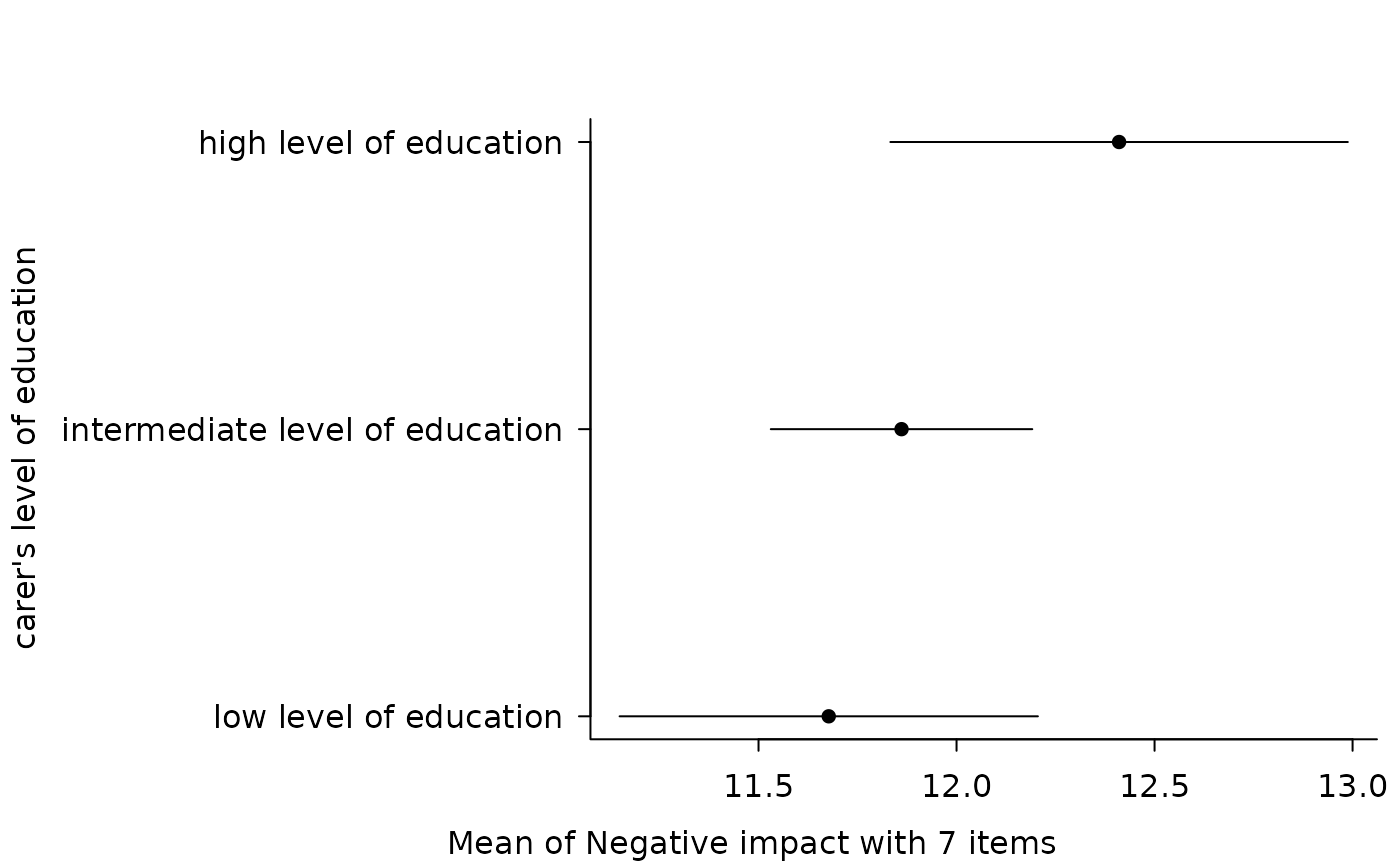# \donttest{ # Customize aesthetics layers <- visualisation_recipe(x, jitter = list(width = 0.03, color = "red"), line = list(linetype = "dashed") ) plot(layers)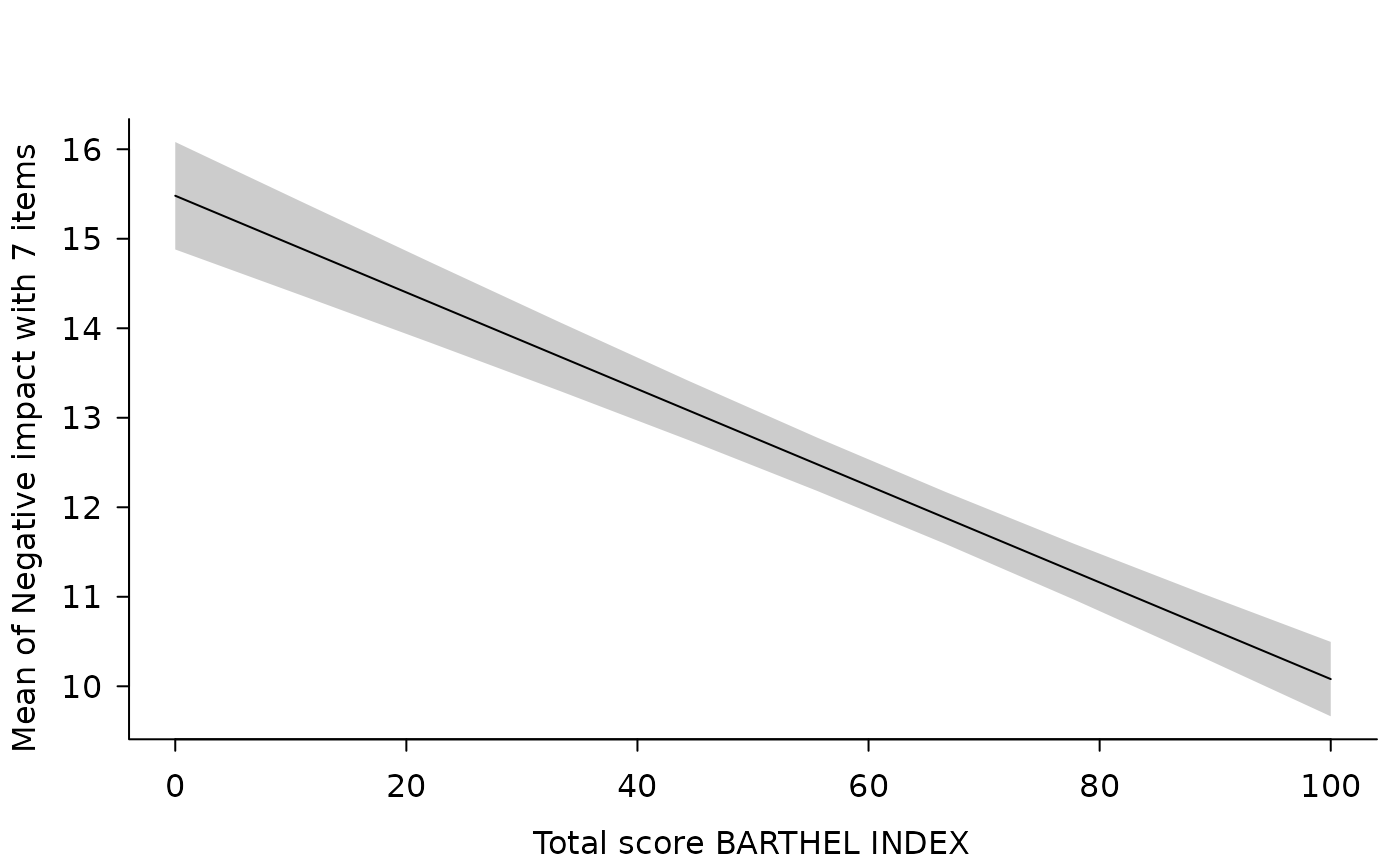# Customize raw data plot(visualisation_recipe(x, show_data = c("violin", "boxplot", "jitter")))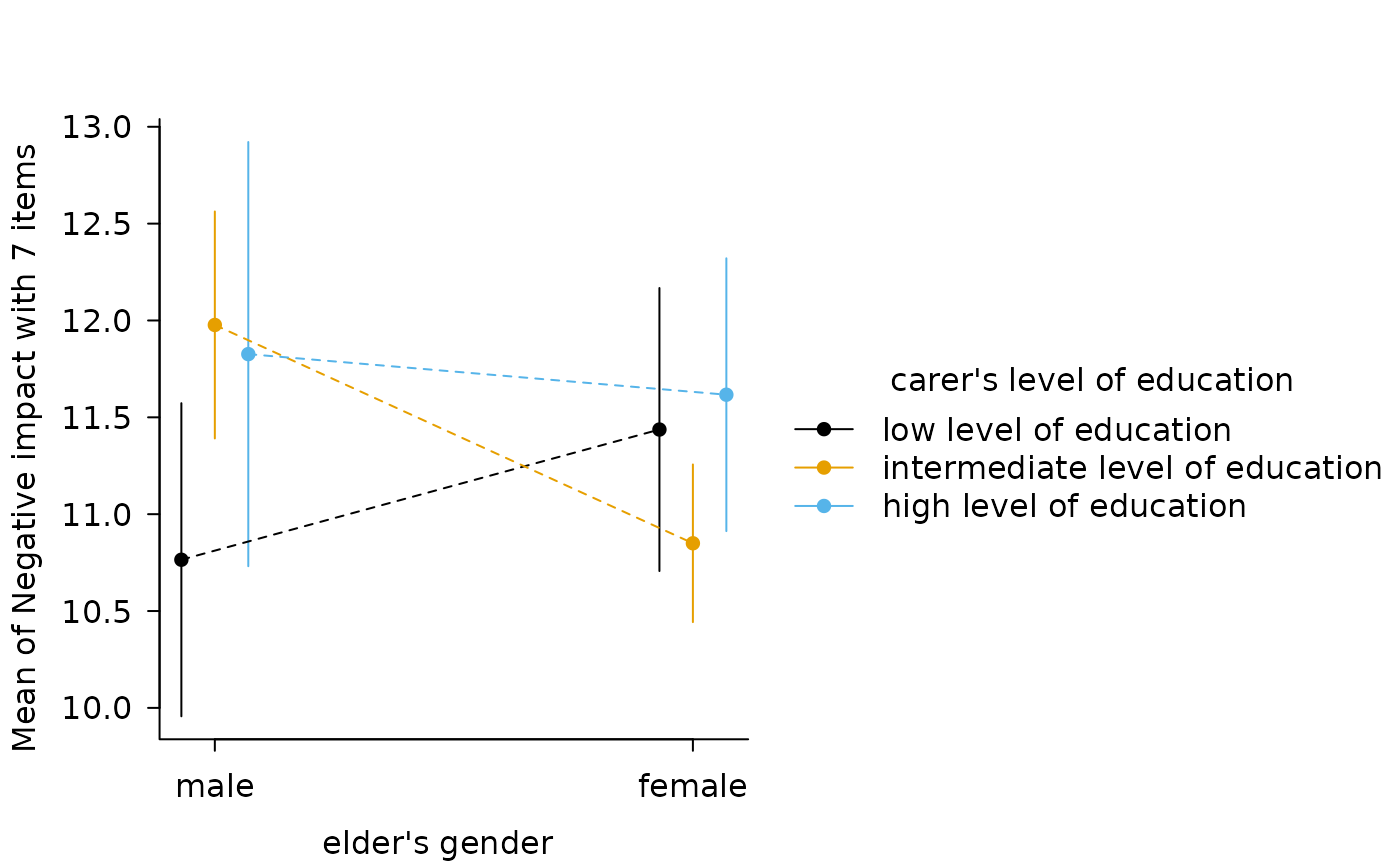# Two levels --------------- data <- mtcars data$cyl <- as.factor(data$cyl) data$new_factor <- as.factor(rep(c("A", "B"), length.out = nrow(mtcars)))

model <- lm(mpg ~ new_factor * cyl * wt, data = data)
x <- estimate_means(model, at = c("new_factor", "cyl"))
#> Warning: Could not recover model data from environment. Please make sure your
#>   data is available in your workspace.
#>   Trying to retrieve data from the model frame now.
#> NOTE: Results may be misleading due to involvement in interactions
#> Warning: Could not recover model data from environment. Please make sure your
#>   data is available in your workspace.
#>   Trying to retrieve data from the model frame now.
plot(visualisation_recipe(x))
#> Warning: Could not recover model data from environment. Please make sure your
#>   data is available in your workspace.
#>   Trying to retrieve data from the model frame now.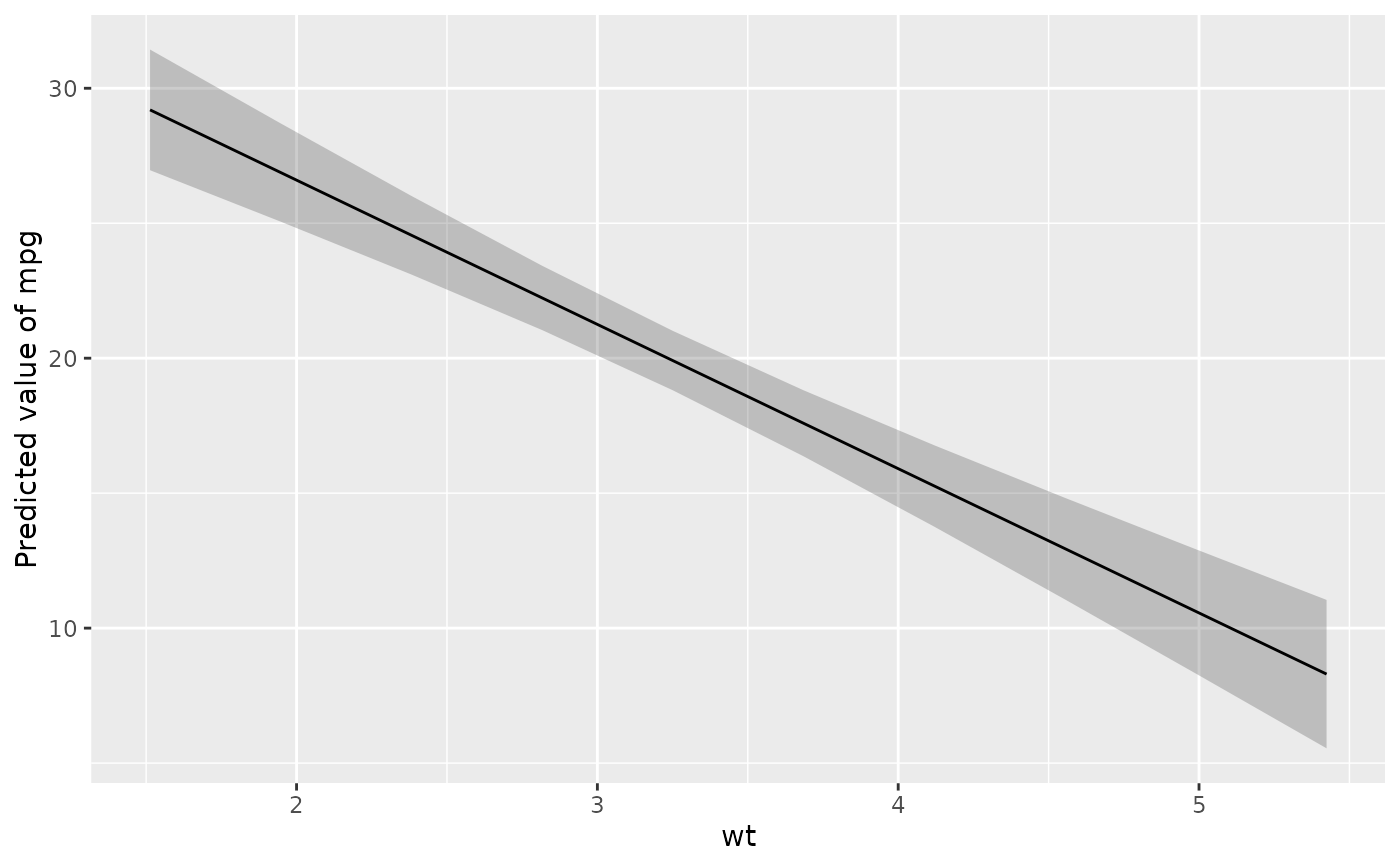# Modulations --------------
x <- estimate_means(model, at = c("new_factor", "wt"))
#> Warning: Could not recover model data from environment. Please make sure your
#>   data is available in your workspace.
#>   Trying to retrieve data from the model frame now.
#> NOTE: Results may be misleading due to involvement in interactions
#> Warning: Could not recover model data from environment. Please make sure your
#>   data is available in your workspace.
#>   Trying to retrieve data from the model frame now.
plot(visualisation_recipe(x))
#> Warning: Could not recover model data from environment. Please make sure your
#>   data is available in your workspace.
#>   Trying to retrieve data from the model frame now.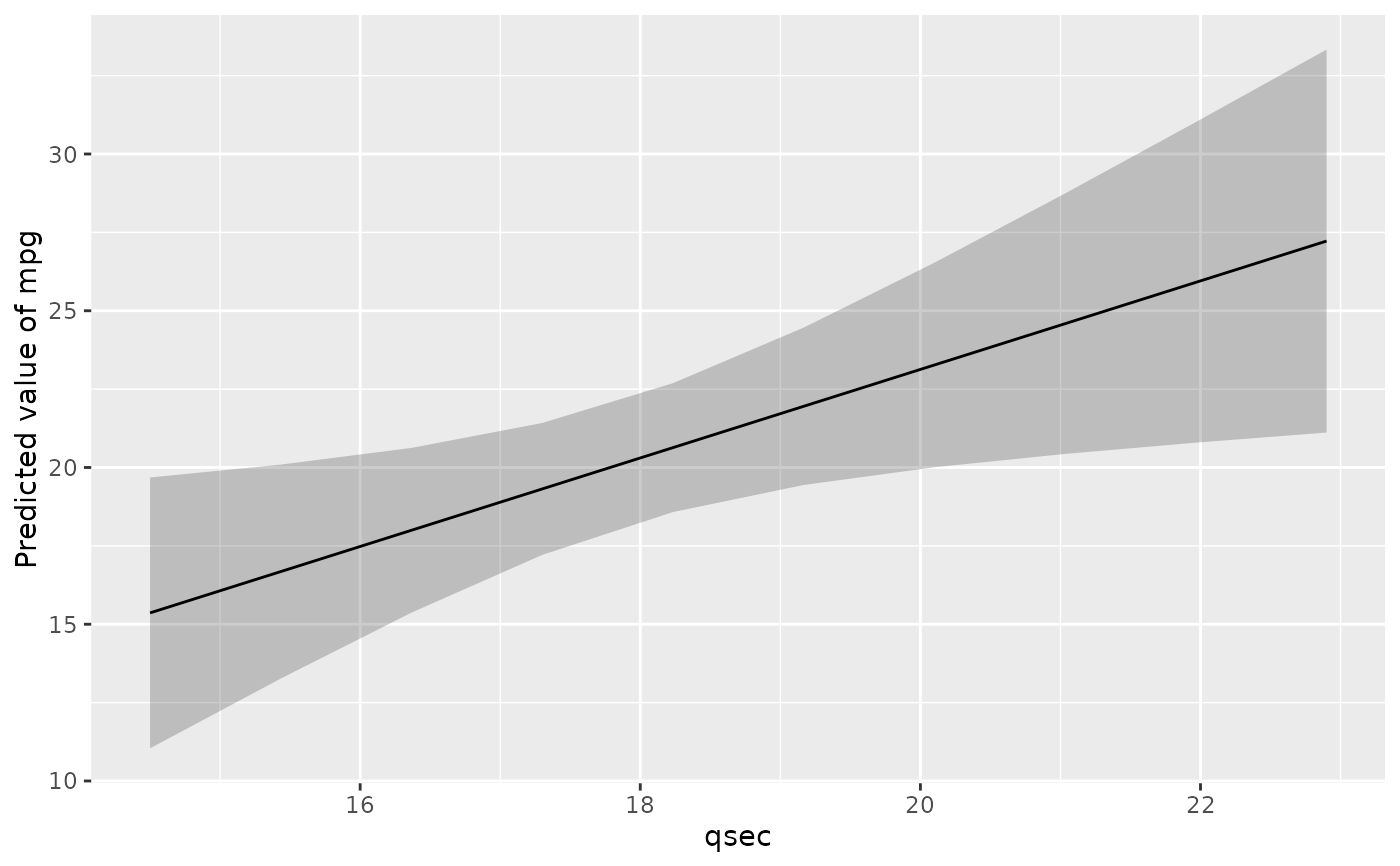x <- estimate_means(model, at = c("new_factor", "cyl", "wt"))
#> Warning: Could not recover model data from environment. Please make sure your
#>   data is available in your workspace.
#>   Trying to retrieve data from the model frame now.
#> Warning: Could not recover model data from environment. Please make sure your
#>   data is available in your workspace.
#>   Trying to retrieve data from the model frame now.
plot(visualisation_recipe(x))
#> Warning: Could not recover model data from environment. Please make sure your
#>   data is available in your workspace.
#>   Trying to retrieve data from the model frame now.
#> Error in geom_from_list(x[[i]]): Problem while computing aesthetics.
#> ℹ Error occurred in the 2nd layer.
#> Caused by error in FUN():

#'   # GLMs ---------------------
data <- data.frame(vs = mtcars$vs, cyl = as.factor(mtcars$cyl))
x <- estimate_means(glm(vs ~ cyl, data = data, family = "binomial"))
#> Warning: Could not recover model data from environment. Please make sure your
#>   data is available in your workspace.
#>   Trying to retrieve data from the model frame now.
#> We selected at = c("cyl").
#> Warning: Could not recover model data from environment. Please make sure your
#>   data is available in your workspace.
#>   Trying to retrieve data from the model frame now.
plot(visualisation_recipe(x))
#> Warning: Could not recover model data from environment. Please make sure your
#>   data is available in your workspace.
#>   Trying to retrieve data from the model frame now.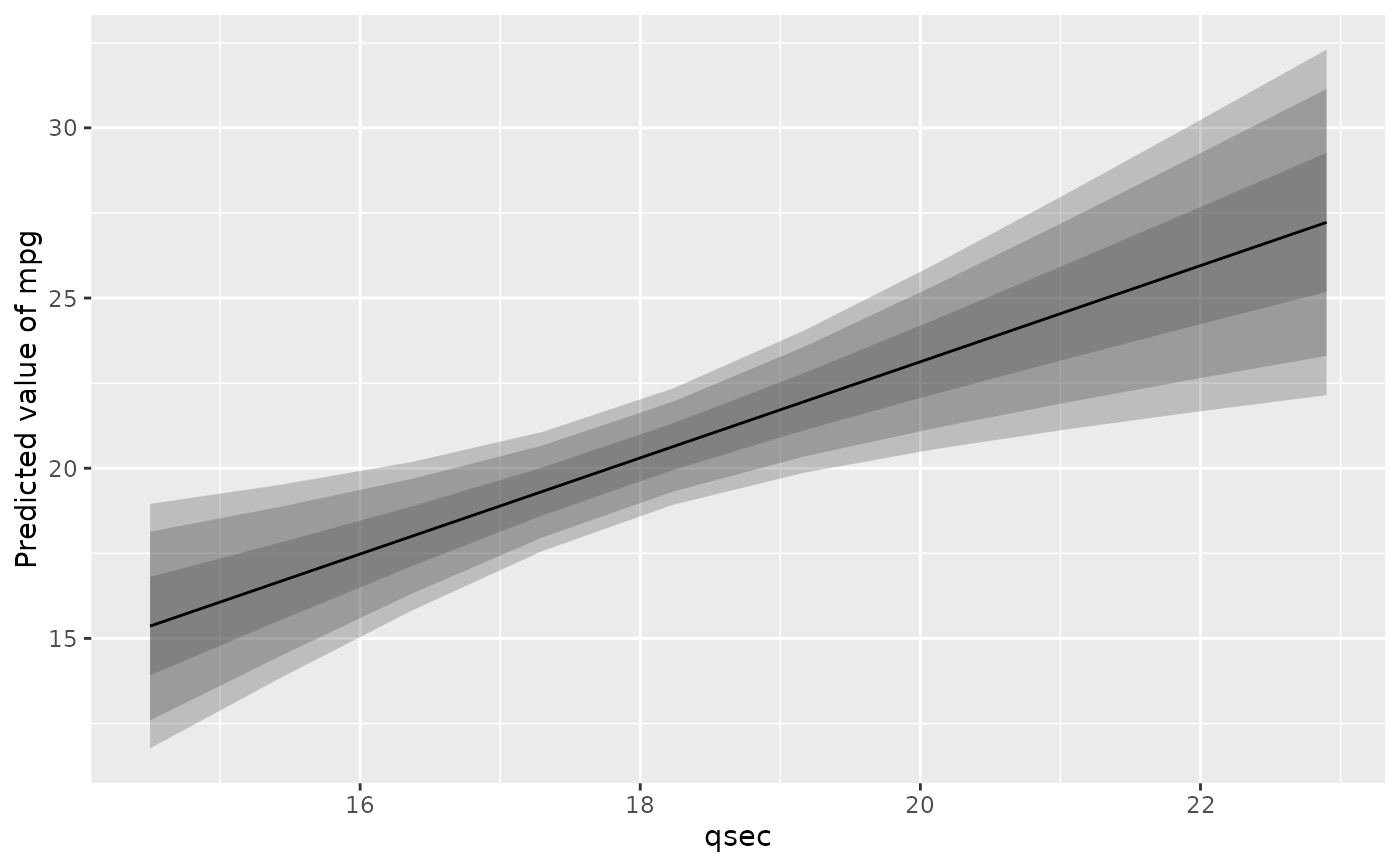# }
# ==============================================
# estimate_relation, estimate_response, ...
# ==============================================
if (require("ggplot2")) {
# Simple Model ---------------
x <- estimate_relation(lm(mpg ~ wt, data = mtcars))
layers <- visualisation_recipe(x)
layers
plot(layers)
}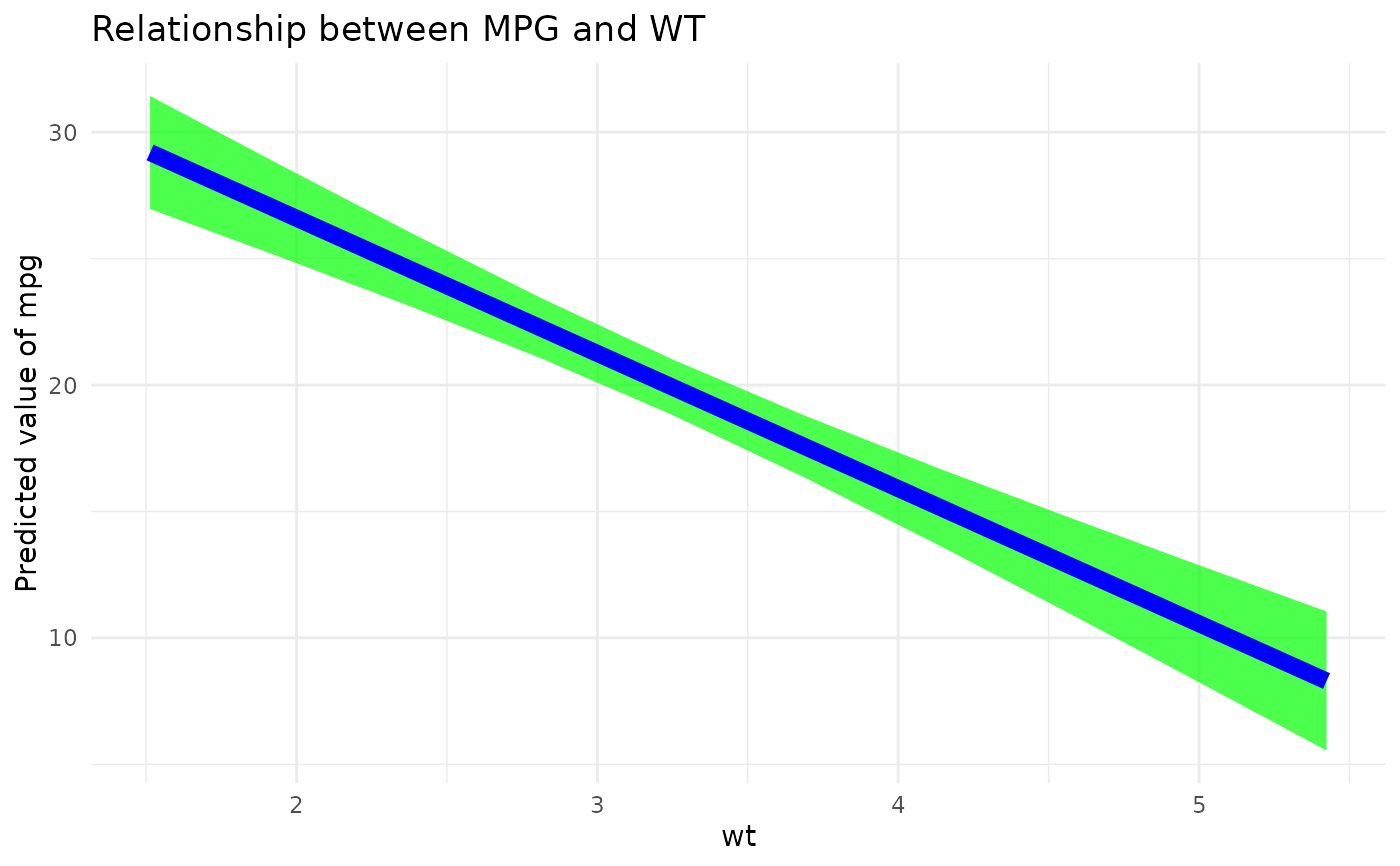# \donttest{
if (require("ggplot2")) {
# Customize aesthetics ----------

layers <- visualisation_recipe(x,
point = list(color = "red", alpha = 0.6, size = 3),
line = list(color = "blue", size = 3),
ribbon = list(fill = "green", alpha = 0.7),
labs = list(subtitle = "Oh yeah!")
)
layers
plot(layers)

# Customize raw data -------------

plot(visualisation_recipe(x, show_data = "none"))
plot(visualisation_recipe(x, show_data = c("density_2d", "points")))
plot(visualisation_recipe(x, show_data = "density_2d_filled"))
plot(visualisation_recipe(x, show_data = "density_2d_polygon"))
plot(visualisation_recipe(x, show_data = "density_2d_raster")) +
scale_x_continuous(expand = c(0, 0)) +
scale_y_continuous(expand = c(0, 0))

# Single predictors examples -----------

plot(estimate_relation(lm(Sepal.Length ~ Sepal.Width, data = iris)))
plot(estimate_relation(lm(Sepal.Length ~ Species, data = iris)))

# 2-ways interaction ------------

# Numeric * numeric
x <- estimate_relation(lm(mpg ~ wt * qsec, data = mtcars))
layers <- visualisation_recipe(x)
plot(layers)

# Numeric * factor
x <- estimate_relation(lm(Sepal.Width ~ Sepal.Length * Species, data = iris))
layers <- visualisation_recipe(x)
plot(layers)

# Factor * numeric
x <- estimate_relation(lm(Sepal.Width ~ Species * Sepal.Length, data = iris))
layers <- visualisation_recipe(x)
plot(layers)

# 3-ways interaction ------------

data <- mtcars
data$vs <- as.factor(data$vs)
data$cyl <- as.factor(data$cyl)
data$new_factor <- as.factor(rep(c("A", "B"), length.out = nrow(mtcars))) # Numeric * numeric * numeric x <- estimate_relation(lm(mpg ~ wt * qsec * hp, data = data), length = c(5, 3, 20)) layers <- visualisation_recipe(x) plot(layers) # Numeric * numeric * factor x <- estimate_relation(lm(mpg ~ wt * am * vs, data = data)) layers <- visualisation_recipe(x) plot(layers) # Numeric * factor * factor x <- estimate_relation(lm(mpg ~ wt * cyl * new_factor, data = data)) layers <- visualisation_recipe(x) plot(layers) # Factor * numeric * numeric x <- estimate_relation(lm(mpg ~ cyl * qsec * hp, data = data)) layers <- visualisation_recipe(x) plot(layers) + scale_size_continuous(range = c(0.2, 1)) # GLMs --------------------- x <- estimate_relation(glm(vs ~ mpg, data = mtcars, family = "binomial")) plot(visualisation_recipe(x)) plot(visualisation_recipe(x, show_data = "jitter", point = list(height = 0.03))) # Multiple CIs --------------------- plot(estimate_relation(lm(mpg ~ disp, data = mtcars), ci = c(.50, .80, .95) )) plot(estimate_relation(lm(Sepal.Length ~ Species, data = iris), ci = c(0.5, 0.7, 0.95) )) } #> Warning: Could not recover model data from environment. Please make sure your #> data is available in your workspace. #> Trying to retrieve data from the model frame now. #> Warning: Could not recover model data from environment. Please make sure your #> data is available in your workspace. #> Trying to retrieve data from the model frame now. #> Warning: Could not recover model data from environment. Please make sure your #> data is available in your workspace. #> Trying to retrieve data from the model frame now. #> Warning: Could not recover model data from environment. Please make sure your #> data is available in your workspace. #> Trying to retrieve data from the model frame now. #> Warning: Could not recover model data from environment. Please make sure your #> data is available in your workspace. #> Trying to retrieve data from the model frame now. #> Warning: Could not recover model data from environment. Please make sure your #> data is available in your workspace. #> Trying to retrieve data from the model frame now. #> Warning: Could not recover model data from environment. Please make sure your #> data is available in your workspace. #> Trying to retrieve data from the model frame now. #> Warning: Could not recover model data from environment. Please make sure your #> data is available in your workspace. #> Trying to retrieve data from the model frame now.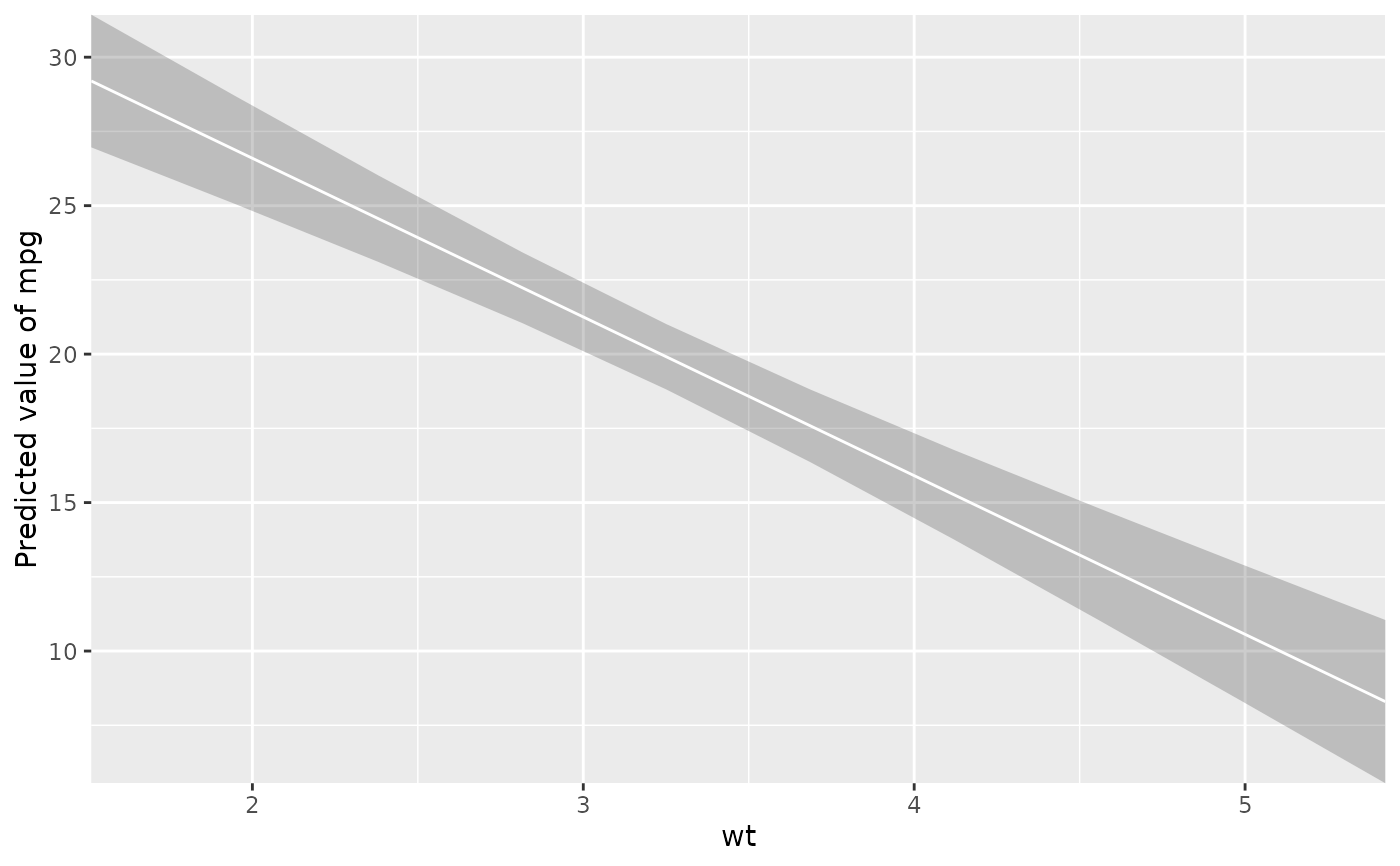# Bayesian models --------------------- if (require("ggplot2") && require("rstanarm")) { model <- rstanarm::stan_glm(mpg ~ wt, data = mtcars, refresh = 0) # Plot individual draws instead of regular ribbon x <- estimate_relation(model, keep_iterations = 100) layers <- visualisation_recipe(x, ribbon = list(color = "red")) plot(layers) model <- rstanarm::stan_glm(Sepal.Width ~ Species * Sepal.Length, data = iris, refresh = 0) plot(estimate_relation(model, keep_iterations = 100)) }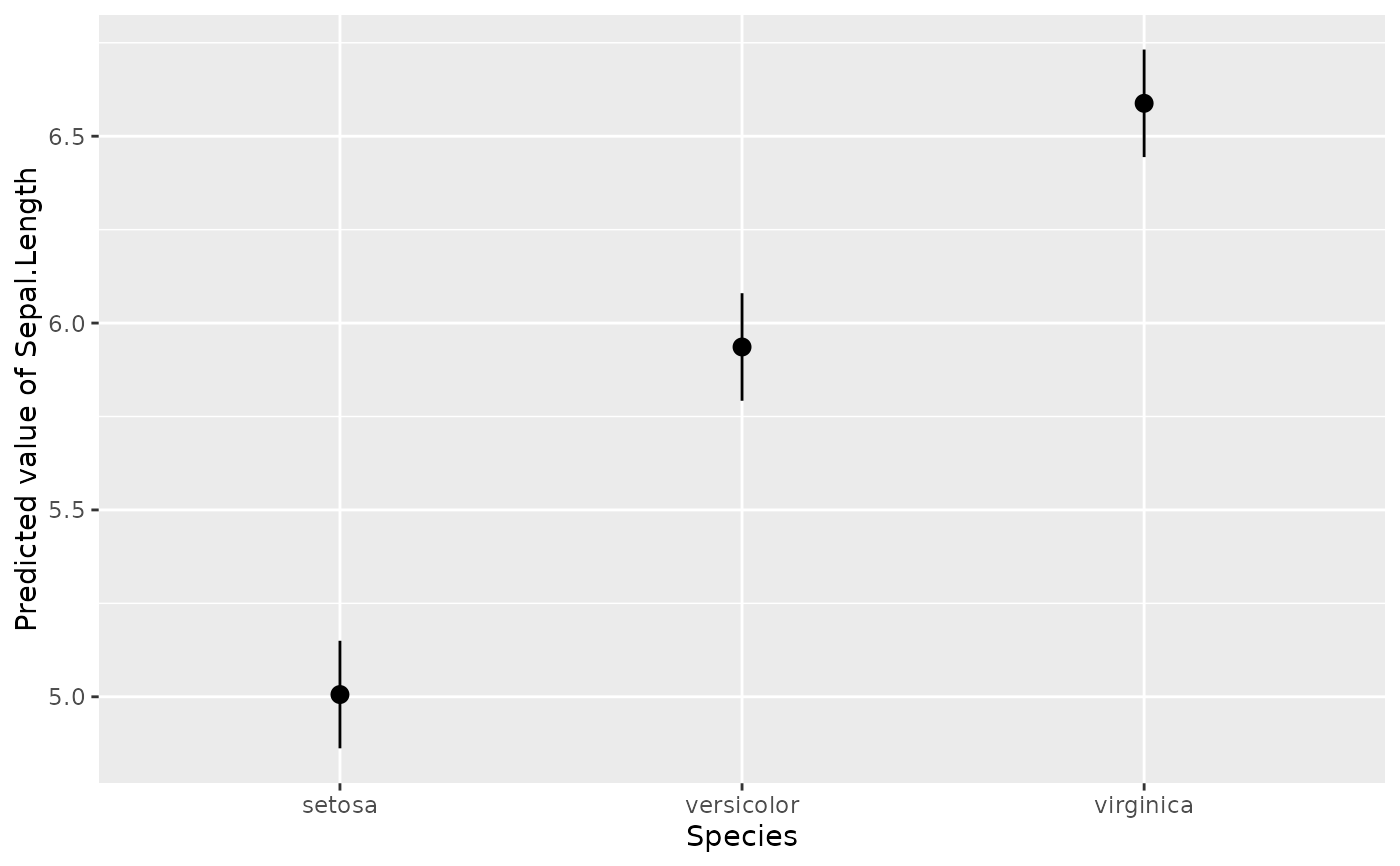# } # ============================================== # estimate_slopes # ============================================== if (require("ggplot2")) { model <- lm(Sepal.Width ~ Species * Petal.Length, data = iris) x <- estimate_slopes(model, trend = "Petal.Length", at = "Species") layers <- visualisation_recipe(x) layers plot(layers) model <- lm(Petal.Length ~ poly(Sepal.Width, 4), data = iris) x <- estimate_slopes(model, at = "Sepal.Width", length = 20) plot(visualisation_recipe(x)) model <- lm(Petal.Length ~ Species * poly(Sepal.Width, 3), data = iris) x <- estimate_slopes(model, at = c("Sepal.Width", "Species")) plot(visualisation_recipe(x)) } #> No numeric variable was specified for slope estimation. Selecting trend = "Sepal.Width". #> No numeric variable was specified for slope estimation. Selecting trend = "Sepal.Width". #> Warning: Using alpha for a discrete variable is not advised.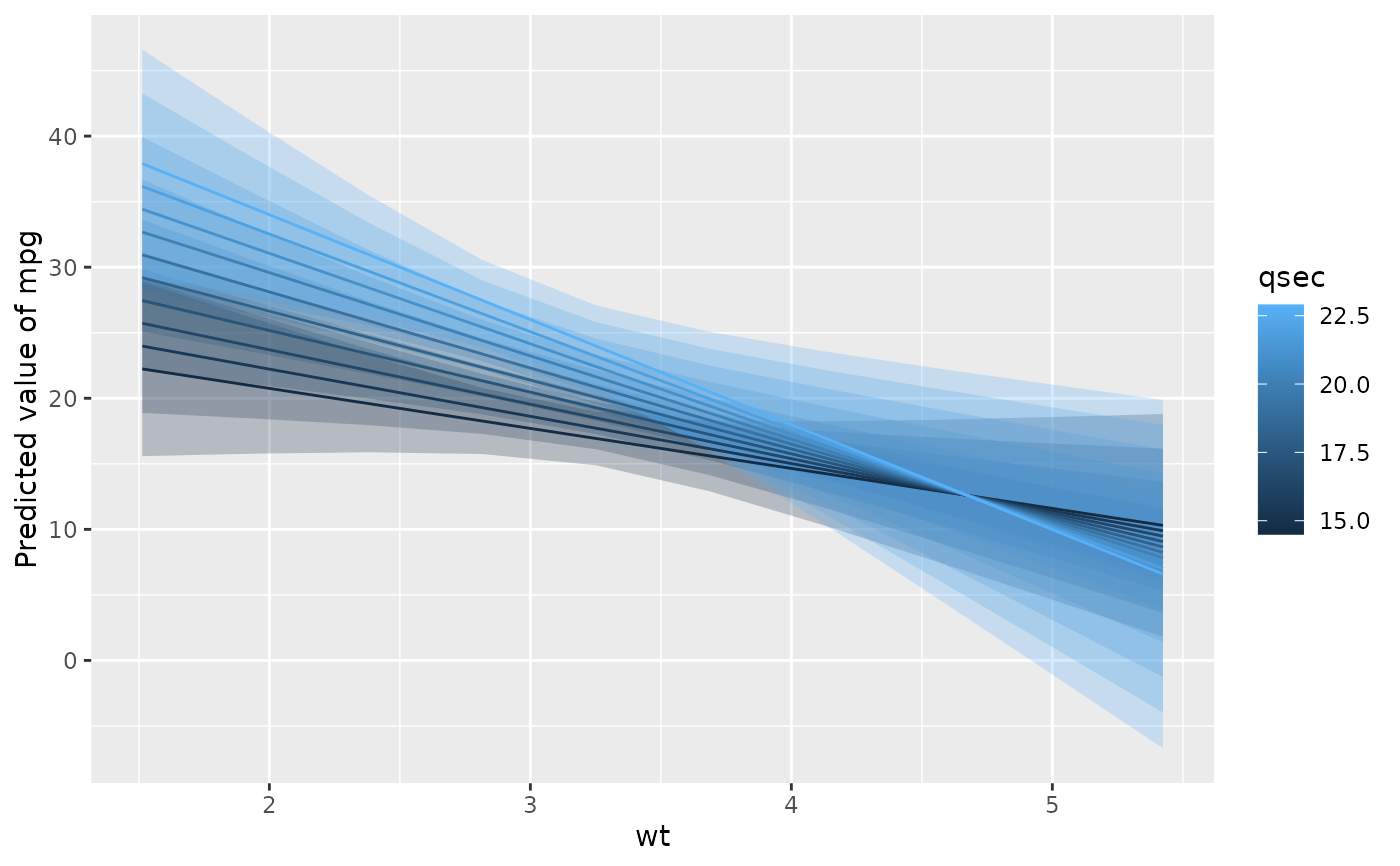# \dontrun{ # TODO: fails with latest emmeans (1.8.0) if (require("mgcv")) { data <- iris data$Petal.Length <- data\$Petal.Length^2

model <- mgcv::gam(Sepal.Width ~ t2(Petal.Width, Petal.Length), data = data)
x <- estimate_slopes(model, at = c("Petal.Width", "Petal.Length"), length = 20)
plot(visualisation_recipe(x))

model <- mgcv::gam(Sepal.Width ~ t2(Petal.Width, Petal.Length, by = Species), data = data)
x <- estimate_slopes(model, at = c("Petal.Width", "Petal.Length", "Species"), length = 10)
plot(visualisation_recipe(x))
}
#> Warning: Could not recover model data from environment. Please make sure your
#>   data is available in your workspace.
#>   Trying to retrieve data from the model frame now.
#> No numeric variable was specified for slope estimation. Selecting trend = "Petal.Width".
#> Warning: Could not recover model data from environment. Please make sure your
#>   data is available in your workspace.
#>   Trying to retrieve data from the model frame now.
#> Warning: Could not recover model data from environment. Please make sure your
#>   data is available in your workspace.
#>   Trying to retrieve data from the model frame now.
#> No numeric variable was specified for slope estimation. Selecting trend = "Petal.Width".
#> Warning: Could not recover model data from environment. Please make sure your
#>   data is available in your workspace.
#>   Trying to retrieve data from the model frame now.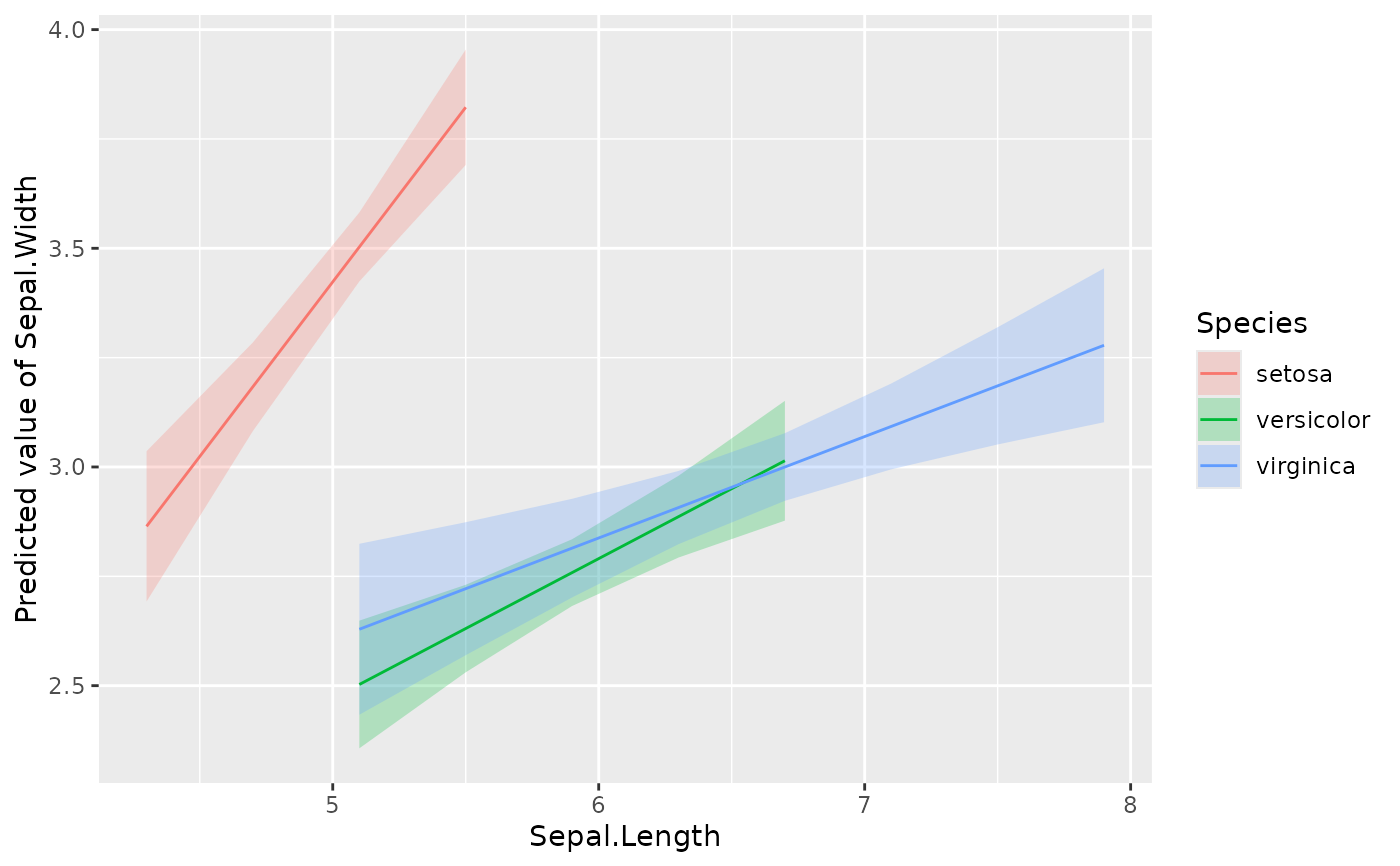# }